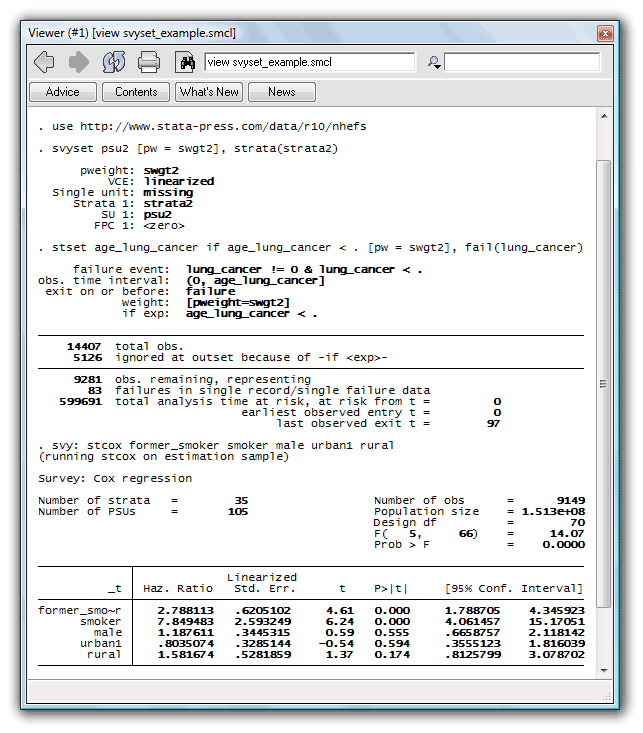Home  /  Products  /  Stata 10  /   Survey and correlated data

## Survey and correlated data

Stata’s svy: prefix now works with

• Cox proportional hazards regression (stcox)
• Parametric hazard and accelerated time survival regression (streg)

Twenty-five other commands also now support estimation with survey data.

You just declare the survey design for your data by using svyset, and then declare your data to be survival-time data by using stset. Here’s an example:

. use http://www.stata-press.com/data/r10/nhefs
. svyset psu2 [pw = swgt2], strata(strata2)
. stset age_lung_cancer if age_lung_cancer < . [pw = swgt2], fail(lung_cancer)
. svy: stcox former_smoker smoker male urban1 ruralWe could just as easily have fitted a parametric survival regression model simply by replacing svy:stcox with svy:streg.

### Here’s a complete list of what’s new in statistics(survey)

1. Stata’s svy: prefix now works with 48 estimators, 27 more than previously. Other commands with which svy: now works include

biprobit bivariate probit regression conditional (fixed effects) logistic regression complementary log-log regression censored-normal regression constrained linear regression generalized linear models heteroskedastic probit regression instrumental-variables regression probit model with endogenous regressors tobit model with endogenous regressors multinomial probit regression nonlinear least-squares estimation
scobit skewed logistic regression stereotype logistic regression Cox proportional hazards regression parametric survival regression (five estimators) tobit regression treatment-effects model truncated regression zero-inflated negative binomial regression zero-inflated Poisson regression zero-truncated negative binomial regression zero-truncated Poisson regression

See [SVY] svy estimation.
2. svy: prefix now calculates the linearized variance estimator two to 100 times faster, the larger multiplier applying to large datasets with many sampling units; see [SVY] svy.
3. svy: mean, svy: proportion, svy: ratio, and svy: total are considerably faster when the over() option identifies many subpopulations.
4. svy:, svy: mean, svy: proportion, svy: ratio, and svy: total now take advantage of multiple processors in Stata/MP, making them even faster.
5. Concerning svyset,

1. New option singleunit(method) provides three methods for handling strata with one sampling unit. If not specified, the default in such cases is to report standard errors as missing value.
2. New option fay(#) specifies that Fay’s adjustment be made to the BRR weights.
See [SVY] svyset.
6. estat has two new subcommands for use with svy estimation results:

1. estat sd, used after svy: mean, reports subpopulation standard deviations.
2. estat strata reports the number of singleton and certainty strata within each sampling stage.
See [SVY] estat.
7. svy: tabulate now allows string variables. See [SVY] svy: tabulate oneway and [SVY] svy: tabulate twoway.
8. Existing command svydes has been renamed svydescribe; svydes continues to work.
svydescribe now puts missing values in the generate(newvar) variable for observations outside the specified estimation sample. Previously, the variable would contain a zero for observations outside the estimation sample. See [SVY] svydescribe.
9. The [SVY] manual has been reorganized. Stata’s survey estimation commands are now documented in [SVY] svy estimation. All model-specific information is now documented in the manual entry for the corresponding estimation command.